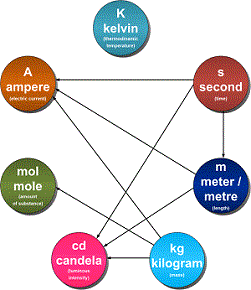# Units and Measurements - Online Test

Q1. Physical quantities are
Explaination / Solution:

A physical quantity is a physical property of a phenomenon, body, or substance, that can be quantified by measurement.A physical quantity can be expressed as the combination of a magnitude expressed by a number – usually a real number – and a unit. All these given above can be expressed as explained so these are physical quantity.

Q2. Measurement of a physical quantity is essentially the
Explaination / Solution:

The Measurement of a given quantity is essentially an act or result of comparison between a quantity whose magnitude (amount) is unknown, with a similar quantity whose magnitude (amount) is known, the latter quantity being called a Standard.

Q3. Fundamental or base quantities are arbitrary. In SI system these are
Explaination / Solution:

A base quantity is chosen and arbitrarily defined, rather than being derived from a combination of other physical quantities. The 7 base quantities are:

PHYSICAL QUANTITYBASE SI UNIT
Mass (m)Kilogram (Kg)
Length (ll)Metre (m)
Time (t)Second (s)
Current (II)Ampere (A)
Temperature (T)Kelvin (K)
Amount of sub. (n)Molar (mol)
Luminous Intensity (L)Candela (cd

Q4. Unit for a fundamental physical quantity is
Explaination / Solution:

Unit is the reference used as the standard measurement of a physical quantity. The unit in which the fundamental quantities are measured are called fundamental unit and the units used to measure derived quantities are called derived units.

Q5. In SI system the fundamental units are
Explaination / Solution:

The SI base units and their physical quantities are the metre for measurement of length, the kilogram for mass, the second for time, the ampere for electric current, the kelvin for temperature, the candela for luminous intensity, and the mole for amount of substance.Q6. Derived units
Explaination / Solution:

Derived units are units which may be expressed in terms of base units by means of mathematical symbols of multiplication and division.

For example, the SI derived unit of area is the square metre (m​​​​​​2), and the SI derived unit of density is the kilogram per cubic metre (kg/m3 or kg m​​​​​​-3).

The names of SI units are written in lowercase.

Q7. Joule is the SI unit of
Explaination / Solution:

The joule (symbol J), is a derived unit of energy in the International System of Units. It is equal to the energy transferred to (or work done on) an object when a force of one newton acts on that object in the direction of its motion through a distance of one metre (1 newton metre or N⋅m).

Q8. Newton is the SI unit of
Explaination / Solution:

The newton is the SI unit for force; it is equal to the amount of net force required to accelerate a mass of one kilogram at a rate of one meter per second squared

Q9. The least count of any measuring equipment is
Explaination / Solution:

The smallest value that can be measured by the measuring instrument is called its least count. Measured values are good only up to this value. The least count error is the error associated with the resolution of the instrument.

Q10. Resolution is
Explaination / Solution:

Resolution is the smallest amount of input signal change that the instrument can detect reliably. This term is determined by the instrument noise (either circuit or quantization noise).